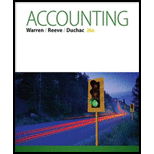Chapter 3, Problem 3.19EX### Accounting (Text Only)

26th Edition
Carl Warren + 2 others
ISBN: 9781285743615

#### Solutions

Chapter
Section### Accounting (Text Only)

26th Edition
Carl Warren + 2 others
ISBN: 9781285743615
Textbook Problem
4 views

# Determining fixed asset’s book valueThe balance in the equipment account is $28,650,000, and the balance in the accumulated depreciation—equipment account is$16,430,000.a. What is the book value of the equipment?b. Does the balance in the accumulated depreciation account mean that the equipment’s loss of value is $16,430,000? Explain. (a) To determine Fixed assets: Fixed assets are long lived economic resources which are owned by the company. Fixed asset accounts are increased by the debits and decreased by the credits. Thus, fixed asset accounts normally show debit balances (balance sheet). Depreciation expenses: Depreciation is permanent decreases in the monetary value of an asset over the time period due to use, wear and tear or obsolescence. It is the process of allocating (allocation concept) the cost of an asset to expense over its useful life. Depreciation expense is the amount of depreciation that is reported (expenses) on the income statement. Accumulated depreciation: Accumulated depreciation refers to the amount of depreciation that is subtracted from the value of assets in the balance sheet over a period of time. The book value of equipment. Explanation The book value of equipment is as follows: The book value of equipment = (Cost ofequipmentAccumulated depreciation)=$28,650,000$16,430,000=$12,220,000

Hence, the book value of equipment is \$12,220,000

(b)

To determine

To explain: Whether the balance of accumulated depreciation indicates the equipment’s loss of value.

### Still sussing out bartleby?

Check out a sample textbook solution.

See a sample solution

#### The Solution to Your Study Problems

Bartleby provides explanations to thousands of textbook problems written by our experts, many with advanced degrees!

Get Started

#### Find more solutions based on key concepts